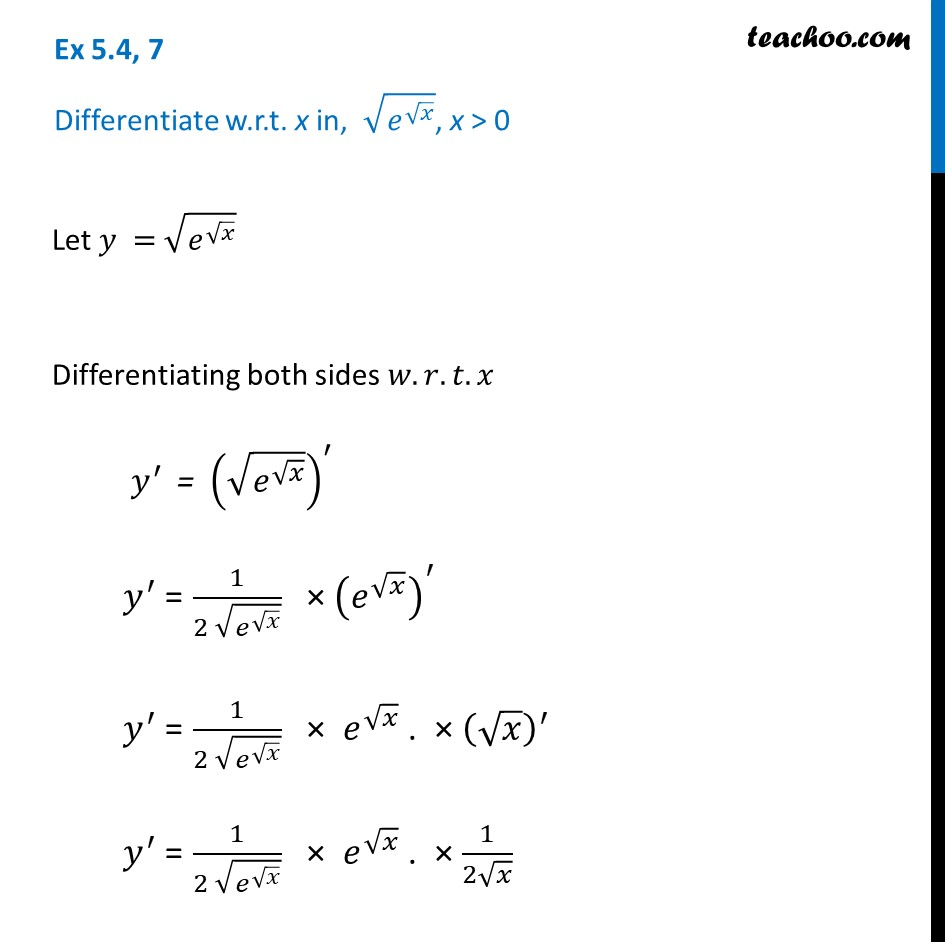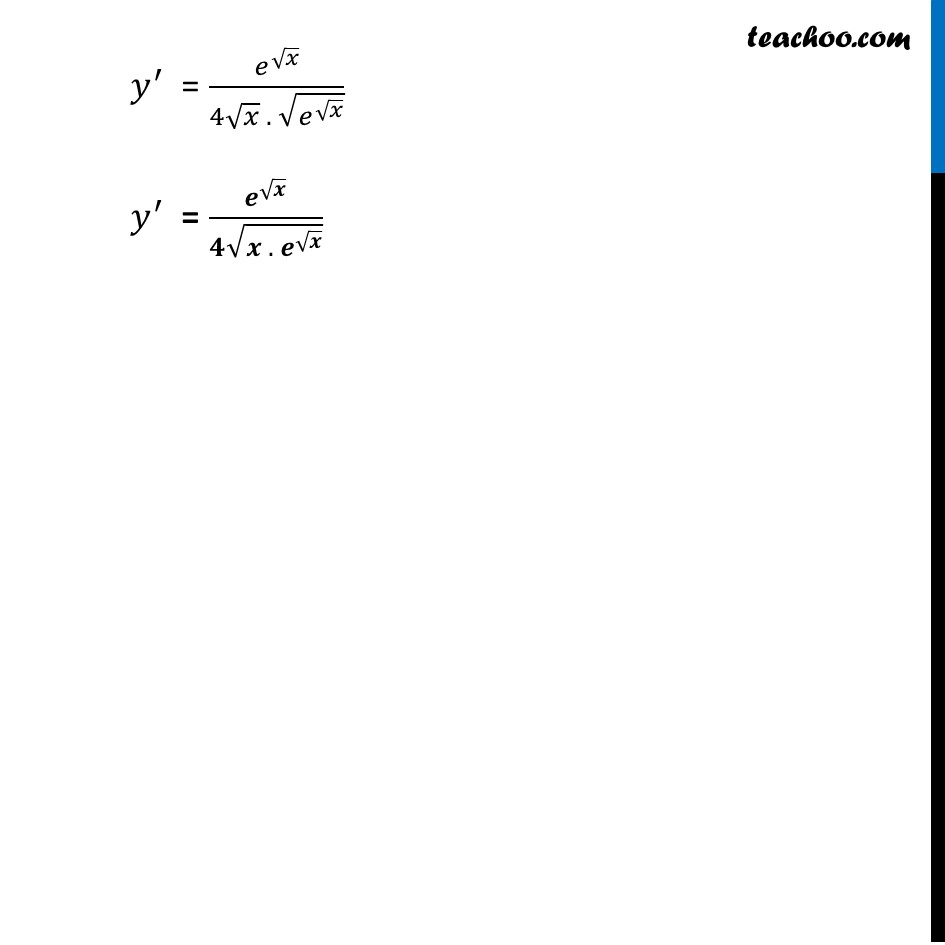Finding derivative of Exponential & logarithm functions

Chapter 5 Class 12 Continuity and Differentiability
Concept wiseLearn in your speed, with individual attention - Teachoo Maths 1-on-1 Class

### Transcript

Ex 5.4, 7 Differentiate w.r.t. x in, √(𝑒^√𝑥 ), x > 0Let 𝑦 = √(𝑒^√𝑥 ) Differentiating both sides 𝑤.𝑟.𝑡.𝑥 𝑦^′ = (√(𝑒^√𝑥 ))^′ 𝑦^′ = 1/(2 √(𝑒^√𝑥 )) × (𝑒^√𝑥 )^′ 𝑦^′ = 1/(2 √(𝑒^√𝑥 )) × 𝑒^√𝑥 . × (√𝑥)^′ 𝑦^′ = 1/(2 √(𝑒^√𝑥 )) × 𝑒^√𝑥 . × 1/(2√𝑥) 𝑦^′ = 𝑒^√𝑥/(4√𝑥 . √(𝑒^√𝑥 )) 𝑦^′ = 𝒆^√𝒙/(𝟒√(𝒙 . 𝒆^√𝒙 ))